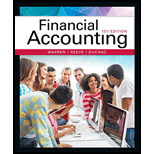# A building with a cost of $1,200,000 has an estimated residual value of$250,000, has an estimated useful life of 40 years, and is depreciated by the straight-line method. (a) What is the amount of the annual depreciation? (b) What is the book value at the end of the twenty-eighth year of use? (c) If at the start of the twenty-ninth year it is estimated that the remaining life is 10 years and that the residual value is $180,000, what is the depreciation expense for each of the remaining 10 years?BuyFindarrow_forward ### Financial Accounting 15th Edition Carl Warren + 2 others Publisher: Cengage Learning ISBN: 9781337272124 #### Solutions Chapter SectionBuyFindarrow_forward ### Financial Accounting 15th Edition Carl Warren + 2 others Publisher: Cengage Learning ISBN: 9781337272124 Chapter 10, Problem 12E Textbook Problem 181 views ## A building with a cost of$1,200,000 has an estimated residual value of $250,000, has an estimated useful life of 40 years, and is depreciated by the straight-line method. (a) What is the amount of the annual depreciation? (b) What is the book value at the end of the twenty-eighth year of use? (c) If at the start of the twenty-ninth year it is estimated that the remaining life is 10 years and that the residual value is$180,000, what is the depreciation expense for each of the remaining 10 years?

(a)

To determine

Determine the amount of annual depreciation.

### Explanation of Solution

Straight-line Depreciation: Under the straight-line method of depreciation, the same amount of depreciation is allocated every year over the estimated useful life of an asset. The formula to calculate the depreciation cost of the asset using the salvage value is shown as below:

Depreciation cost = (Original cost of the asset-residual value)Estimated useful life of the asset

Determine the amount of annual depreciation.

Cost of the equipment =$1,200,000 Estimated residual Value =$250,000

Estimated useful life =40 years

AnnualD

(b)

To determine

Determine the book value at the end of the twenty-eighth year of use.

(c)

To determine

Determine the revised annual depreciation for each of the remaining 10 years.

### Still sussing out bartleby?

Check out a sample textbook solution.

See a sample solution

#### The Solution to Your Study Problems

Bartleby provides explanations to thousands of textbook problems written by our experts, many with advanced degrees!

Get Started

Find more solutions based on key concepts
If a stock is not in equilibrium, explain how financial markets adjust to bring it into equilibrium.

Fundamentals of Financial Management, Concise Edition (MindTap Course List)

Why do executives and managers spend more time listening than do workers?

Essentials of Business Communication (MindTap Course List)

(Cooperatives) How do cooperatives differ from typical businesses?

ECON: MICRO4 (New, Engaging Titles from 4LTR Press)

STATEMENT OF STOCKHOLDERS' EQUITY Computer World Inc. paid out 22.5 million in total common dividends and repor...

Fundamentals of Financial Management, Concise Edition (with Thomson ONE - Business School Edition, 1 term (6 months) Printed Access Card) (MindTap Course List)